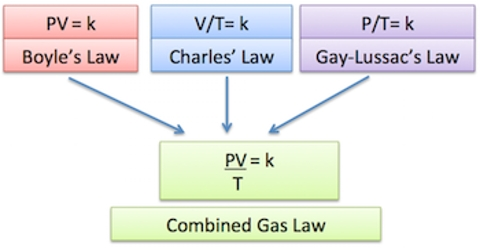# Use of the Gas Equation in Calculations Involving Gases

The Use of the Gas Equation in Calculations Involving Gases

Various types of calculations using the ideal gas equation are possible. For this purpose, the equation may be used in different forms as shown below. Combining Charles’s Law, Boyle’s Law, and Gay-Lussac’s Law gives us the combined gas law. For a gas with constant molar mass, the three other state variables are interrelated. Here g is the mass of a given quantity of gas, M is the molecular mass and ρ is the density of the gas.

(a) PV = nRT (Ideal Gas Equation)

(b) PV = (g/M) RT

(c) P = (g/V) x (RT/M) = ρ (RT/M)

(d) (P1V1)/T1 = (P2V2)/T2

The units of pressure that are used are pascal (Pa), standard atmosphere (atm), and torr.  1 atm is the average pressure at sea level.  It is normally used as a standard unit of pressure.  The SI unit though is the pascal. 101,325 pascals equals 1 atm.